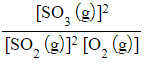# Edexcel A Level Chemistry:复习笔记1.10.4 Deducing Kc Expressions

### Deducing Kc Expressions

#### Equilibrium expression & constant

• The equilibrium expression links the equilibrium constant, Kc, to the concentrations of reactants and products at equilibrium taking the stoichiometry of the equation into account
• So, for a given reaction:

aA + bB ⇌ cC + dD

• Kc is defined as follows: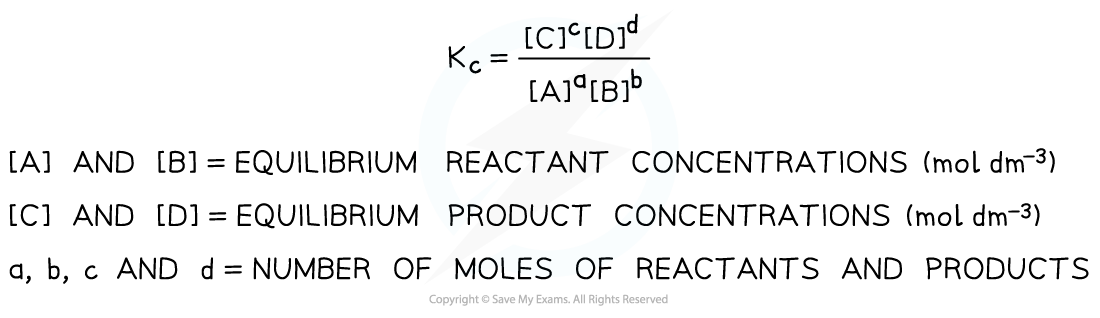Equilibrium expression linking the equilibrium concentration of reactants and products at equilibrium

• The Kc of a reaction is specific and only changes if the temperature of the reaction changes

#### Homogeneous systems and Kc

• A homogeneous system is where all of the reactants and products are in the same physical state, e.g.

CH3COOH (l) + C2H5OH (l) ⇌ CH3COOC2H5 (l) + H2O (l)

• For this reaction, all of the reactants and products are in the same, liquid state / phase and will, therefore, all feature in the Kc expression
• Kc =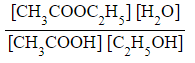#### Heterogeneous systems and Kc

• A heterogeneous system is where not all of the reactants and products are in the same physical state, e.g.

CaCO3 (s) ⇌ CaO (s) + CO2 (g)

• Solids are ignored in equilibrium expressions
• This leads to a Kc expression of Kc = [CO2]

#### Exam Tip

For Kc expressions, it is important that you use square brackets as sometimes examiners are instructed to be strict about the appearance of brackets in expressions

Square brackets implies concentration

#### Worked Example

Deducing equilibrium expressions

Deduce the equilibrium expression for the following reactions:

1. Ag+ (aq) + Fe2+ (aq) ⇌ Ag (s) + Fe3+ (aq)
2. N2 (g) + 3H2 (g) ⇌ 2NH3 (g)
3. 2SO2 (g) + O2 (g) ⇌ 2SO3 (g)

• Kc =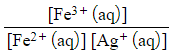• [Ag (s)] is not included in the equilibrium expression as it is a solid

• Kc =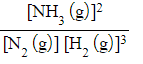• Kc =